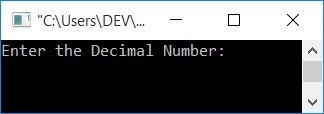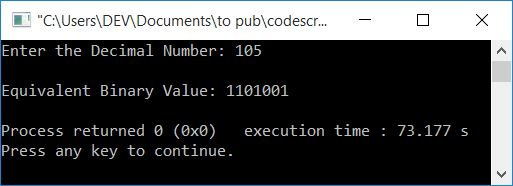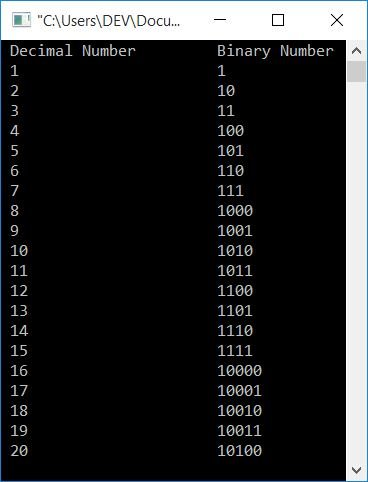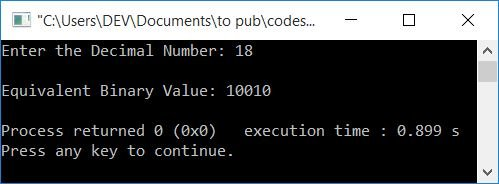# C++ Program to Convert Decimal to Binary

In this article, you will learn about and get C++ code for decimal to binary conversion. The program is created using the following approaches:

Some extra programs are also available. However, before going through all of the programs available here, if you are unfamiliar with the steps used for the conversion, you can refer to Decimal to Binary to get everything you need.

## Decimal to Binary using an Array in C++

To convert a decimal number to a binary number in C++ programming, you have to ask the user to enter the decimal number first. and then convert it into its equivalent binary value. Print its equivalent binary value on the output as shown in the program given below.

The question is: write a program in C++ to receive a decimal number and print its equivalent value in binary. The answer to this question is given below:

```#include<iostream>
using namespace std;
int main()
{
int decimalNum, binaryNum, i=0;
cout<<"Enter the Decimal Number: ";
cin>>decimalNum;
while(decimalNum!=0)
{
binaryNum[i] = decimalNum%2;
i++;
decimalNum = decimalNum/2;
}
cout<<"\nEquivalent Binary Value: ";
for(i=(i-1); i>=0; i--)
cout<<binaryNum[i];
cout<<endl;
return 0;
}```

This program was built and runs under the Code::Blocks IDE. Below is a sample run:Now supply any decimal number input, say 105, and press the ENTER key to print its equivalent value in the binary number system as shown in the output given below:The dry run of the above program with user input 105 (a decimal number) goes like this:

1. Initial value, i=0
2. When the user enters a decimal number, say 105, then it gets stored in decimalNum. So decimalNum=105
3. The while loop's condition decimalNum!=0 is now evaluated.
4. The condition decimalNum!=0 or 105!=0 is evaluated to be true, therefore program flow goes inside the loop.
5. And decimalNum%2 or 105%2 or 1 gets initialized to binaryNum[i] or binaryNum. So, binaryNum=1
6. The value of i gets incremented. Now i=1
7. And decimalNum/2 or 52 is set to decimalNum. Now decimalNum=52
8. Program flow goes back and evaluates the condition of the while loop again with a new value of 52.
9. Because the condition again evaluates to being true, the program flow again goes inside the loop.
10. And again, decimalNum%2 or 52%2 or 0 gets initialized to binaryNum[i] or binaryNum. So binaryNum=0
11. Again the value of i gets incremented. So i=2
12. After that, decimalNum/2, 52/2, or 26 is initialized to decimalNum. So decimalNum=26
13. Process the same, from step no. 8 to 12 with the new value of decimalNum and i.
14. On continuing the process in a similar way until the condition of the while loop evaluates to be false, we will get the values of binaryNum[] as:
• binaryNum=1
• binaryNum=0
• binaryNum=0
• binaryNum=1
• binaryNum=0
• binaryNum=1
• binaryNum=1
15. When the value of decimalNum becomes equal to 0, then the condition decimalNum!=0 or 0!=0 evaluates to be false, and therefore the evaluation of the while loop gets ended. The value of binaryNum[] is then printed using the for loop.
16. The value of binaryNum[] is printed from the last to the 0th index.
17. That will be 1101001

### From 1 to 20, convert decimal to binary

Here is another program that converts all the decimal numbers from 1 to 20 to their equivalent binary values.

```#include<iostream>
using namespace std;
int main()
{
int decimalNum, binaryNum, i, k;
cout<<" Decimal Number\t\tBinary Number\n";
for(k=1; k<=20; k++)
{
i=0;
decimalNum = k;
while(decimalNum!=0)
{
binaryNum[i] = decimalNum%2;
i++;
decimalNum = decimalNum/2;
}
cout<<" "<<k<<"\t\t\t";
for(i=(i-1); i>=0; i--)
cout<<binaryNum[i];
cout<<endl;
}
cout<<endl;
return 0;
}```

Here is the output produced by the above program:## Decimal to Binary Conversion without Array

The question is: write a program in C++ that converts a decimal number to a binary number without using an array. Here is its answer. This program doesn't use an array to convert any given number in decimal to its equivalent binary value.

```#include<iostream>
using namespace std;
int main()
{
int decimalNum, binaryNum=0, mul=1, rem;
cout<<"Enter the Decimal Number: ";
cin>>decimalNum;
while(decimalNum>0)
{
rem = decimalNum%2;
binaryNum = binaryNum+(rem*mul);
mul = mul*10;
decimalNum = decimalNum/2;
}
cout<<"\nEquivalent Binary Value: "<<binaryNum;
cout<<endl;
return 0;
}```

Here is a sample run with 18 as a decimal number as user input:### Decimal to binary conversion in C++, using a user-defined function

This program also does the same job as the previous program. The only difference is that this program was created using a user-defined function, DecimalToBinary(). That is, this function was created to convert decimal to binary.

This function takes a decimal number as its argument and returns its equivalent binary value. The return value is initialized to binaryNum (in the main() function). Print the value of "binaryNum" as output.

```#include<iostream>
using namespace std;
int DecimalToBinary(int);
int main()
{
int decimalNum, binaryNum;
cout<<"Enter the Decimal Number: ";
cin>>decimalNum;
binaryNum = DecimalToBinary(decimalNum);
cout<<"\nEquivalent Binary Value: "<<binaryNum;
cout<<endl;
return 0;
}
int DecimalToBinary(int decimalNum)
{
int binaryNum=0, mul=1, rem;
while(decimalNum>0)
{
rem = decimalNum%2;
binaryNum = binaryNum+(rem*mul);
mul = mul*10;
decimalNum = decimalNum/2;
}
return binaryNum;
}```

It will produce the same output as the previous program. You can also use user-defined functions to create the same purpose program:

```#include<iostream>
using namespace std;
int DecimalToBinary(int, int);
int binaryNum;
int main()
{
int decimalNum, i;
cout<<"Enter the Decimal Number: ";
cin>>decimalNum;
i = DecimalToBinary(decimalNum, 0);
cout<<"\nEquivalent Binary Value: ";
for(i=(i-1); i>=0; i--)
cout<<binaryNum[i];
cout<<endl;
return 0;
}
int DecimalToBinary(int decimalNum, int i)
{
while(decimalNum!=0)
{
binaryNum[i] = decimalNum%2;
i++;
decimalNum = decimalNum/2;
}
return i;
}```

#### The same program in different languages

C++ Quiz

« Previous Program Next Program »